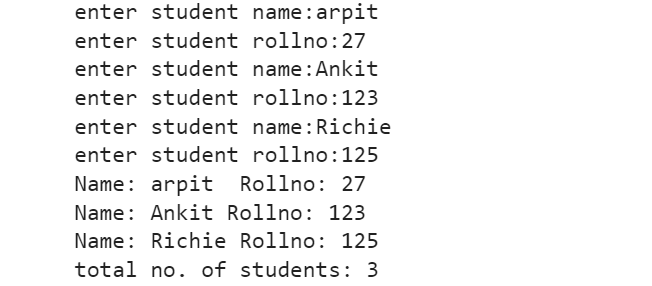# Using a Class with Input in Python

• Last Updated : 20 Feb, 2022

In this article, we will see how to take input using class in Python.

## Using a Class with Input in Python

It is to be noted that while using class in Python, the __init__() method is mandatory to be called for declaring the class data members, without which we cannot declare the instance variable (data members) for the object of the class. A variable declared outside the __init__() methods is termed as the class variable such as stuCount variable in the below program. It is to be noted that the class variable is accessed by className.classVariableName, e.g., Student.StuCount in the above program. However, the instance variables or data members are accessed as self.instanceVariableName.

## Python

 `# Illustration of creating a class ` `# in Python with input from the user ` `class` `Student: ` `    ``'A student class'` `    ``stuCount ``=` `0` ` `  `    ``# initialization or constructor method of ` `    ``def` `__init__(``self``):   ` `         `  `        ``# class Student ` `        ``self``.name ``=` `input``(``'enter student name:'``) ` `        ``self``.rollno ``=` `input``(``'enter student rollno:'``) ` `        ``Student.stuCount ``+``=` `1` ` `  `    ``# displayStudent method of class Student ` `    ``def` `displayStudent(``self``):   ` `        ``print``(``"Name:"``, ``self``.name, ``"Rollno:"``, ``self``.rollno) ` ` `  ` `  `stu1 ``=` `Student() ` `stu2 ``=` `Student() ` `stu3 ``=` `Student() ` `stu1.displayStudent() ` `stu2.displayStudent() ` `stu3.displayStudent() ` `print``(``'total no. of students:'``, Student.stuCount) `

Output:In this program, we see that the input() function is called in the definition of __init__() method for inputting the values of data variables name and rollno. Subsequently, the value of stuCount is incremented by 1 in order to keep track of the total number of objects created for the class Student. In this program, three objects stu1, stu2, and stu3 are created thereby calling the constructor function __init__() three times. The values are assigned to name and rollno after input from the user. Then, the result is displayed by calling the displayStudent() function of class Student. The output of this program can be preferred for more lucidity.

My Personal Notes arrow_drop_up
Recommended Articles
Page :